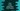# How to create a string from ASCII values in Dart## Dart program to create a string from ASCII values:

The full form of ASCII is American Standard Code for Information Interchange. It is a character encoding standard for electronic communication(wikipedia). For example, the ASCII value of A is 65 and Z is 90.

You may face scenarios where you need to build the string from a list of ASCII values in your Flutter app. For example, if the server is sending the data in ASCII format, you need to convert it to a user-readable format. Or, you can convert a string to a list of ASCII values and send that to the server. Both of these can be achieved easily in Dart.

Let’s learn how to convert a list of ASCII values to a string and vice versa.

### Example 1: By using String.fromCharCode() method:

We can use the fromCharCode method defined in the String class to convert a ASCII value to a string. If the ASCII values are given in a list, we can iterate over the list items and build the final string.

``````void main() {
List<int> input = [
72,
101,
108,
108,
111,
32,
87,
111,
114,
108,
100,
33,
33
];
String result = "";

for (int i in input) {
result += String.fromCharCode(i);
}

print("Given String: " + result);
}``````

In this example, input is the given list of ASCII values. We used a for loop to iterate over the numbers of the list and appended the decoded string to the final string result.

If you run this program, it will print:

### Example 2: By using String.fromCharCodes() method:

We can also use the String.fromCharCodes method, which takes an array of values and returns the final string. This is a better approach than the previous one because we don’t have to run a loop to iterate over the characters.

``````void main() {
List<int> input = [
72,
101,
108,
108,
111,
32,
87,
111,
114,
108,
100,
33,
33
];
String result = String.fromCharCodes(input);

print("Given String: " + result);
}``````

It will print:

``Given String: Hello World!!``

### Example 3: Converting a string to a list of ASCII values:

The codeUnitAt method of dart string takes one index as its parameter and returns the ASCII value at that index. We can iterate over the string indices and append all ASCII values of all the indices to get the final list of ASCII values.

``````void main() {
String inputStr = "Hello World!!";
List<int> result = [];

for (int i = 0; i < inputStr.length; i++) {
}

print("Final list: " + result.toString());
}``````

Here, result is the final list of integers. The for loop is iterating over the indices of the string and adds the ASCII values of each character to the final list.

It will print:

``Final list: [72, 101, 108, 108, 111, 32, 87, 111, 114, 108, 100, 33, 33]``

### Example 4: codeUnits property of string:

There is also another property called codeUnits that returns a list of code units of the string. We can use this property directly instead of using a loop to get the list of ASCII values.

``````void main() {
String inputStr = "Hello World!!";
List<int> result = inputStr.codeUnits;

print("Final list: " + result.toString());
}``````

It will print:

``Final list: [72, 101, 108, 108, 111, 32, 87, 111, 114, 108, 100, 33, 33]``